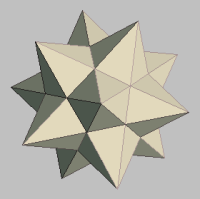MATHGOTSERVEDWelcome to the Accuplacer Math Test Preparation center. Here you will find a wealth of resources that will increase your odds of passing the Accuplacer Math Placement test. There are three sections for the Accuplacer Math test series which are 1) Arithmetic 2) Elementary Algebra, and 3) College Level Mathematics. Below are a collection of links that will help you prepare for the Accuplacer math test.

# Accuplacer Arithmetic Interactive Practice Tests

Please wait for the test to load below....

# About the Accuplacer Arithmetic Section

According to the college board  The Arithmetic algebra "test measures your ability to perform basic arithmetic operations and to solve problems that involve fundamental arithmetic concepts. There are 17 questions on the Arithmetic tests, divided into three types.
• Operations with whole numbers and fractions: Topics included in this category are addition, subtraction, multiplication, division, recognizing equivalent fractions and mixed numbers, and estimating.
• Operations with decimals and percents: Topics include addition, subtraction, multiplication, and division with decimals. Percent problems, recognition of decimals, fraction and percent equivalencies, and problems involving estimation are also given.
• Applications and problem solving: Topics include rate, percent, and measurement problems; simple geometry problems; and distribution of a quantity into its fractional parts." -Source collegeboard.com